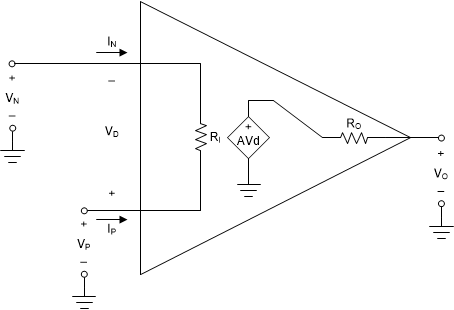SLOA011B January   2018  – July 2021

1. 1Introduction
2. 2Non-Inverting Amplifier
3. 3Inverting Amplifier
4. 4Simplified Op Amp Circuit Diagram
5. 5Op Amp Specifications
6. 6References
7. 7Glossary
8. 8Revision History

## 1.2 Ideal Op Amp Model

The Thevenin amplifier model shown in Figure 1-1 is redrawn in Figure 1-2 showing standard op amp notation. An op amp is a differential to single-ended amplifier. It amplifies the voltage difference, Vd = Vp - Vn, on the input port and produces a voltage, Vo, on the output port that is referenced to ground.Figure 1-2 Standard Op Amp Notation

We still have the loading effects at the input and output ports as noted above. The ideal op amp model was derived to simplify circuit calculations and is commonly used by engineers in first-order approximation calculations. The ideal model makes three simplifying assumptions:

• Gain is infinite
Equation 1. a = ∞
• Input resistance is infinite
Equation 2. Ri = ∞
• Output resistance is zero
Equation 3. Ro = 0

Applying these assumptions to Figure 1-2 results in the ideal op amp model shown in Figure 1-3.Figure 1-3 Ideal Op Amp Model

Other simplifications can be derived using the ideal op amp model:

Equation 4. → In = Ip = 0

Because Ri = ∞, we assume In = Ip = 0. There is no loading effect at the input.

Equation 5. → Vo = a Vd

Because Ro = 0 there is no loading effect at the output.

Equation 6. → Vd = 0

If the op amp is in linear operation, V0 must be a finite voltage. By definition Vo = Vd × a. Rearranging, Vd = Vo / a . Since a = ∞, Vd = Vo / ∞ = 0. This is the basis of the virtual short concept.

Equation 7. Common mode gain = 0

The ideal voltage source driving the output port depends only on the voltage difference across its input port. It rejects any voltage common to Vn and Vp.

Equation 8. Bandwidth = ∞
Equation 9. Slew Rate = ∞

No frequency dependencies are assumed.

Equation 10. Drift = 0

There are no changes in performance over time, temperature, humidity, power supply variations, etc.